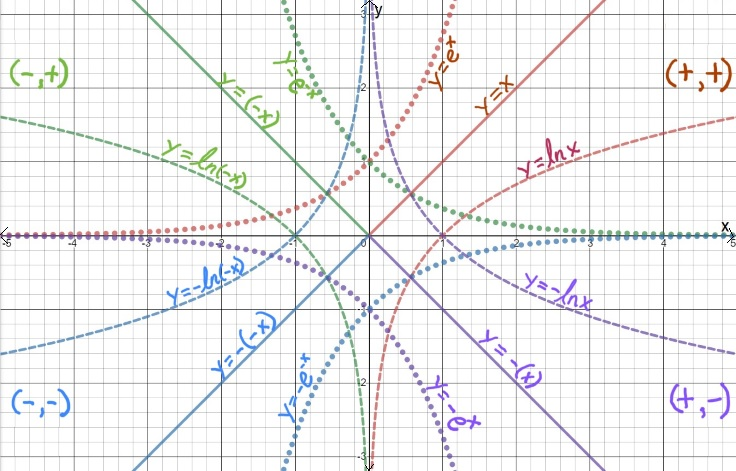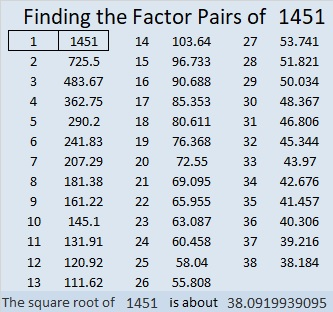# 1451 Star of Wonder

Contents

### Today’s Puzzle:

An important part of Mathematics is noticing patterns. I love it when mathematicians ask students, “What do you notice? What do you wonder?”

Do those questions puzzle you? They are questions you can ponder as you gaze on this star of wonder made from several different graphs.To help distinguish the graphs, the dotted lines are exponential functions, the dashed lines are natural logarithm functions, and the solid lines are linear functions.

What do you notice? What do you wonder?

### Factors of 1451:

Now I’ll tell you a little bit about the post number, 1451:

• 1451 is a prime number.
• Prime factorization: 1451 is prime.
• 1451 has no exponents greater than 1 in its prime factorization, so √1451 cannot be simplified.
• The exponent in the prime factorization is 1. Adding one to that exponent we get (1 + 1) = 2. Therefore 1451 has exactly 2 factors.
• The factors of 1451 are outlined with their factor pair partners in the graphic below.How do we know that 1451 is a prime number? If 1451 were not a prime number, then it would be divisible by at least one prime number less than or equal to √1451. Since 1451 cannot be divided evenly by 2, 3, 5, 7, 11, 13, 17, 19, 23, 29, 31, or 37, we know that 1451 is a prime number.

### More about the Number 1451:

1451 is the sum of two consecutive numbers:
726 + 725 = 1451.

1451 is also the difference of two consecutive squares:
726² – 725² = 1451.

What do you notice about those two facts?

This site uses Akismet to reduce spam. Learn how your comment data is processed.